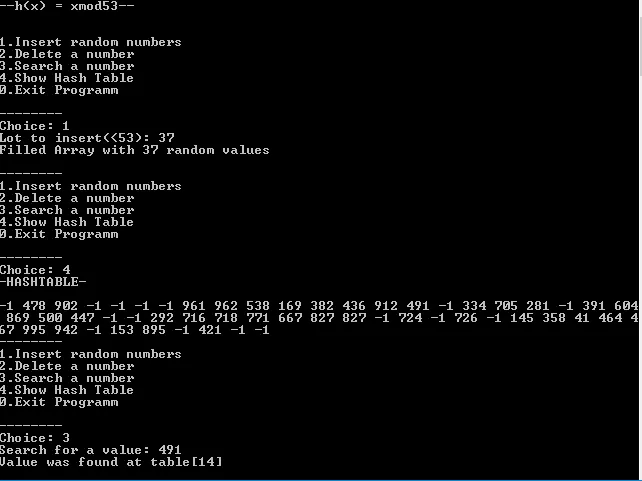# Programming - C Hashtables with Linear ProbingIt's me again with the second part for Hashing! The last part is here and you should read it first to understand some things better, cause here I will only implement Linear Probing in C. I will also explain what needs to be changed to implement another Open Address Method directly! So, let's get started!

# Linear Probing Implementation:

It's pretty easy to implement this type of a Hashtable. It's a simple Array of specific "prime" size and we will insert the values in the hashindex or the next available space if a collision happens. I think the Code will explain itself!

``#include <stdio.h>``
``#include <stdlib.h>``
``#include <string.h>``
``#define N 53``
``#define h(x) (x % m) //h(x) = x mod m``

``// function declarations``
``void fill_array(int *A, int table_range, int number);``
``void insert_table(int *table, int table_range, int hash_index, int val);``
``void print_table(int*table, int table_range);``
``int search_table(int *table, int table_range, int val);``
``void delete(int *table, int table_range, int val);``

``int main(){``
`` ``
``    int *table; // hashtable``
``    int i, j, n, choice, index;``
``    int search, val;``
``    int num = 0; // insert count (I don't check deletions)``

``    printf("--h(x) = xmod%d--\n", N);``
``    printf("\n\n");``
`` ``
``    table = (int*)malloc(N*sizeof(int));``
``    // initialize to -1, value that represents emptyness``
``    for(i = 0; i < N; i++){``
``     table[i] = -1; ``
``    }``
`` ``
``    while(1){``
`` printf("1.Insert random numbers\n");``
`` printf("2.Delete a number\n");``
`` printf("3.Search a number\n");``
`` printf("4.Show Hash Table\n");``
`` printf("0.Exit Programm\n");        ``
`` printf("\n--------\n");``
`` printf("Choice: ");``
`` scanf("%d", &choice);``
`` ``
`` switch(choice){``
``     case 0: exit(0);        ``
``     case 1:``
``         // insert random numbers``
``         do{``
``             printf("Lot to insert(<%d): ", N-num);``
``             scanf("%d", &n);``
``         }while(N - num < n);``
``             ``
``         num += n;``
``         fill_array(table, N, n);``
``         printf("Filled Array with %d random values\n", n);``
``         printf("\n--------\n");``
``         break;``
``     case 2:``
``         // delete a number``
``         printf("Give a value you want to delete: ");``
``         scanf("%d",&search);``
``         delete(table, N, search);``
``         printf("\n--------\n");``
``         break;``
``     case 3: ``
``         // search for a number``
``         printf("Search for a value: ");``
``         scanf("%d",&search);``
``         index=search_table(table, N, search); ``
``         if(index == -1){``
``             printf("This value doesn't exist on HashTable!\n");``
``         }``
``         else{``
``             printf("Value was found at table[%d]\n", index);``
``         }``
``         printf("\n--------\n");``
``         break;``
``     case 4:``
``         //print hashtable``
``         printf("-HASHTABLE-\n\n");  ``
``         print_table(table, N);``
``         printf("\n--------\n");``
``         break;``
``              }``
``    }``
``    return 0;``
``}``

``// FUNCTIONS``
``void fill_array(int *A, int table_range, int number){``
``    int i;``
``    int num;``
``    for(i=0; i<number; i++){``
`` num = rand()%1000; // random number from 0 to 999``
`` insert_table(A, table_range, num % table_range, num);``
``    }``
``}``

``void insert_table(int *table, int table_range, int hash_index, int val){``
`` ``
``    if(table[hash_index] == -1 && hash_index < table_range){``
``     table[hash_index] = val;``
``     return;``
``    }``
``    // go to the next index using modulo when it is outside of the array``
``    hash_index = (hash_index+1) % table_range;``
`` ``
``    if(hash_index == val % table_range){``
`` printf("Hashtable is full!\n");``
`` return;``
``    }``
``    insert_table(table, table_range, hash_index, val);   ``
``}``

``void print_table(int *table, int table_range){``
``    int i;``
``    for(i=0;i<table_range;i++){``
`` printf("%d ",table[i]);``
``    }``
``}``

``int search_table(int *table, int table_range, int val){``
``    int i;``
``    i = val % table_range;``
``    // we search the whole array starting from the hashindex``
``    // cause some value in between could have been deleted``
``    // and we then would have false results``
``    do{``
`` if(table[i] == val){``
``     return i;``
`` }``
`` i = (i+1) % table_range;``
``    }while(i != val % table_range);  ``
``    return -1;``
``}``

``void delete(int *table, int table_range, int val){``
``    int index;``
``    // we call the search function to get the index``
``    index = search_table(table, table_range, val);``
``    if(index == -1){``
``     printf("Value %d doesn't exist on HashTable!\n", val);``
`` return;``
``    }``
``    table[index] = -1;``
``    printf("Value %d was found at table[%d] and was deleted!\n", val, index);``
``}``

An example run would look like this:# How to implement the other Methods:

To implement the others we only have to change this one line!

``    hash_index = (hash_index+1) % table_range;``

When quadratic probing we will have to put it inside of a for loop and starting going in quadratic steps like that:

``hash_index = ((hash_index) + i^2 ) % table_range;``

Because my function is recursive I would have to put the i value as a parameter, starting it out with 0 so that we check the normal hash_index first!

So, the insertion using Quadratic Probing would look like this:

``void insert_table(int *table, int table_range, int hash_index, int val, int i){``
`` ``
`` if(table[hash_index] == -1 && hash_index < table_range){``
``     table[hash_index] = val;``
``     return;``
`` }``
`` hash_index = ((hash_index) + i^2 ) % table_range;``
`` ``
`` if(hash_index == val % table_range){``
``     printf("Hashtable is full!\n");``
``     return;``
`` }``
`` insert_table(table, table_range, hash_index, val);  ``
``}``

Double Hashing is easy! We will have to put not i^2 but i*the second hashfunction. That way we end up with Double Hashing.

The Insertion Function for Double hashing looks like this:

``void insert_table(int *table, int table_range, int hash_index, int val, int i){``
`` if(table[hash_index] == -1 && hash_index < table_range){``
``     table[hash_index] = val;``
``     return;``
`` }``
``     hash_index = (hash_index + i*(val % H)) % table_range;``
`` ``
`` if(hash_index == val % table_range){``
``     printf("Hashtable is full!\n");``
``     return;``
`` }``
`` insert_table(table, table_range, hash_index, val);  ``
``}``

Where H should be another value like N, that is smaller than N, but again a prime number!

This is actually it! Hope you enjoyed all that Hashing stuff!

Next time in Programming we will get into Graphs in Java Implementation!

Bye!

H2
H3
H4
3 columns
2 columns
1 column
1 Comment